# Euclidean Geometry

• Last Updated : 24 Nov, 2021

Geometry word comes from “Geo” which means earth and “meterin” which means to measure. It appears that geometry originated from the need to measure land. It has been studied in almost every civilization for example Egypt, China, India, Greece, etc. Egyptian people were able to calculate simple areas and volumes. They even knew the formula for calculating the volume of a truncated pyramid. Our country also studied geometry in detail in our ancient civilization.

### Geometry in India

In India, the Indus Valley Civilization (about 3000 BCE) made extensive use of geometry. It was a highly organized society. The cities were very well planned. For Instance, roads were parallel and there was present an underground drainage system. It can be concluded that the town dwellers were really skilled in mensuration.

Attention reader! All those who say programming isn't for kids, just haven't met the right mentors yet. Join the  Demo Class for First Step to Coding Coursespecifically designed for students of class 8 to 12.

The students will get to learn more about the world of programming in these free classes which will definitely help them in making a wise career choice in the future.

Same kind of knowledge was found in Sulbasutras, there were manuals of constructions. The Vedic Period’s geometry originated with the construction of fireplaces and altars for performing Vedic rites. For household rituals, squares, circular altars were preferred, and altars having shapes like Triangles, Rectangles, trapeziums were used for public worship. The triangles were arranged in a way that they form Forty-three subsidiary triangles.

### Euclid’s Definition of Geometry

These ancient civilizations used geometry mostly for practical purposes and there was little emphasis on the reasoning behind the statements. Euclid thought of geometry as some abstract model of the world. The idea of the point, lines, shapes were derived from what was seen around in the real world. He studied real-world objects to formalize the concept of solid.

A solid has shape, size, position and can be moved from one place to another. Its boundaries are called surfaces. They separate one part of space from another. They are assumed to have no thickness. The boundaries of surfaces are made up of curves or straight lines. These lines or curves are in turn made up of points.

From solid to the line, at each step, we lose one extension which is defined as dimension.

Euclid’s definitions:

1. A point is that which has no part.
2. A line is breadth less.
3. The ends of a line are points.
4. A straight line is a line that lies evenly with the points on itself.
5. A surface is that which has length and breadth only.
6. The edges of a surface are lines.
7. A plane surface is a surface that lies evenly with straight lines on itself.

In these definitions, there are some terms that are not defined or maybe need further explanations. For example A point.

Mathematicians decide to leave some things undefined. But we do have intuitive feelings of what these terms mean. So, in geometry, we take a point, line, and plane as undefined terms.

Taking these definitions as the base, Euclid assumed some properties which were not to be proved. These assumptions are actually universal truths. They can be divided into two types:

1. Postulates: Assumptions specific to geometry
2. Axioms: Common notions

### Euclid’s Axioms

1. Things which are equal to the same thing are equal to one another.
2. If equals are added to equals, the wholes are equal.
3. If equals are subtracted from equals, the remainders are equal.
4. Things which coincide with one another are equal to one another.
5. The whole is greater than the part.
6. Things which are double of the same things are equal to one another.
7. Things which are halves of the same things are equal to one another.

Now let’s discuss Euclid’s Postulates,

### Euclid’s Postulates

Postulate 1

A straight line may be drawn from any one point to any other point.

Notice that this postulate says that there is at least one straight line that passes through two points but says nothing about whether more than one line like this is possible. But in his work, Euclid has often assumed it to be unique.

Let’s write this result as an axiom

Axiom: Given two distinct points, there is a unique line that passes through them.

This statement is self-evident, let’s see it through a figure.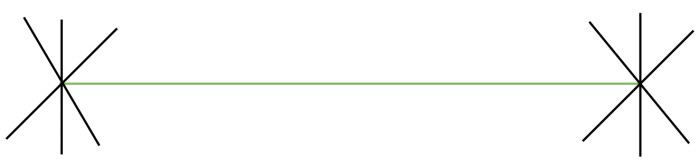Postulate 2

A terminated line can be produced indefinitely.

Euclid terms a line segment as a terminated line. We know that a line segment can be extended in any of the directions.

Postulate 3

A circle can be drawn with any centre and any radius.

Postulate 4

All right angles are equal to one another.

Postulate 5

If a straight line falling on two straight lines makes the interior angles on the same side of it taken together less than two right angles, then the two straight lines, if produced indefinitely, meet on that side on which the sum of angles is less than two right angles.

For example: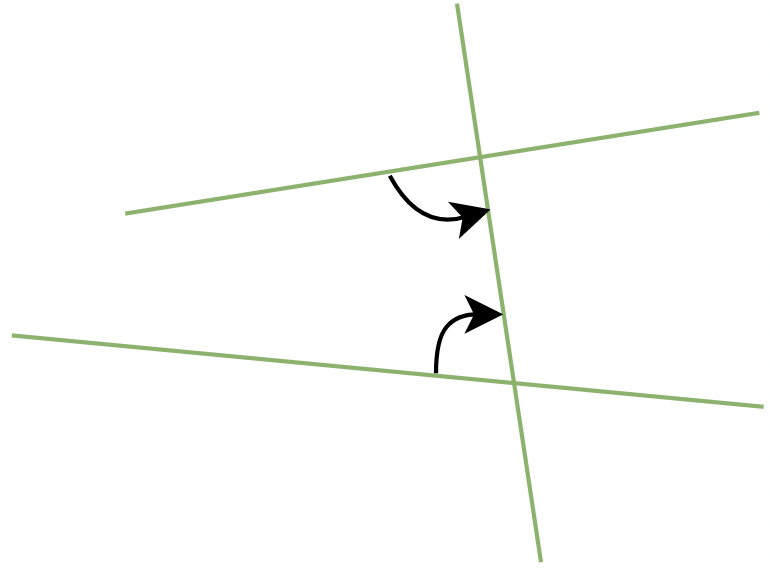We can see here, that the sum of interior angles is less than two right angles. Therefore, these two lines will intersect at a particular point if extended indefinitely.

Postulate 5 is more complex than other postulates. But it is not possible to prove any of them.

Nowadays, ‘postulates’ and ‘axioms’ are used interchangeably and in the same sense. When we say “let us postulate”, it means, “let’s make some statement based on the observed phenomenon in the Universe”. After that its truth or validity is checked. If it passes that test, it is accepted as a postulate.

A system of axioms is called consistent if it is impossible to deduce from these axioms a statement that contradicts any axiom or previously proved statement. So, whenever a system of axioms is given, it is necessary to check for consistency.

After postulates and axioms, Euclid used these to prove other results using deductive reasoning. The statements that were proved are called propositions or theorems.

Theorem: Two distinct lines cannot have more than one point in common.

Proof: Let us assume that there are two distinct lines “m” and “l”. We need to prove that they cannot intersect at more than two points.

For now, consider that these two lines intersect at two points P and Q. So now we have two lines which passing through two distinct points, but it cannot be true as it violates the axiom we studied earlier. So it is not true and our assumption is false.

Thus, two distinct lines cannot have more than one point in common. Hence, Proved.

### Sample Problems on Euclid’s Geometry

Question 1: How many Euclid’s Postulates exist? State each one of them.

There are 5 postulates in Euclid’s Geometry,

1. A straight line may be drawn from any one point to any other point.
2. A terminated line can be produced indefinitely.
3. A circle can be drawn with any centre and any radius.
4. All right angles are equal to one another.
5. If a straight line falling on two straight lines makes the interior angles on the same side of it taken together less than two right angles, then the two straight lines, if produced indefinitely, meet on that side on which the sum of angles is less than two right angles.

Question 2: Define the following terms,

1. Parallel lines
2. Perpendicular lines
3. Line Segment

1. Parallel Lines: The lines that maintain always equal distance from each other and hence they never meet.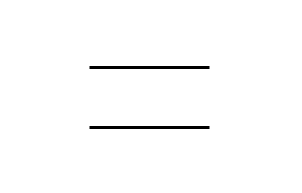Lines: A line is a breadth less length.

Point: A point has no part

2. Perpendicular Lines: When two lines are perpendicular to each other (90° to each other), they are known as perpendicular lines.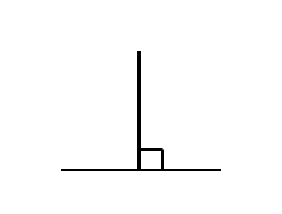Angle: Two lines emerging from a same point form an angle.

3. Line Segment: A Line having two ends and is finite in nature is a Line Segment.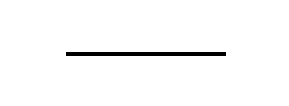4. Radius of a Circle: The Radius of a circle is defined as a line starting from the centre to the circumference.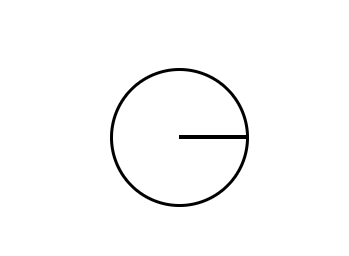Question 3: On a straight lines AB, there exists a point C such that, AC=BC. Prove that the line AC is half of the line segment AB.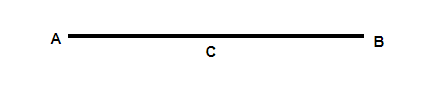Given: AC= BC

To Prove: AC= 1/2 AB

Proof:

We already know from the figure, AB=AC+ BC

But it is given that AC= BC

Therefore, we can write, AB= AC+ AC

AB= 2AC

AC= 1/2 AB

Hence, Proved.

Question 4: In the figure below, AD is a line segment, and B and C are two points lying on the line such that AC = BD, Prove that AB = CD.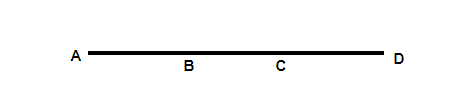Given: AC = BD

To Prove: AB = CD

Proof:

From the given figure, we can write, AC = AB + BC ⇢(1)

BD = BC + CD ⇢(2)

Equating equation (1) and (2) since, AC = BD (given)

AB + BC= BC + CD

AB = CD

Hence, Proved

My Personal Notes arrow_drop_up